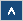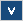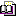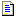Verilog Online HelpTable of ContentsBit-selectBlock StatementsBuilt-in PrimitivesCase StatementContinuous AssignmentsConversion FunctionsCommentsCompiler DirectivesConcatenationsConditional OperatorDelaysDisable StatementDisplay TasksEdge Sensitive PathExpression Bit LengthFile I/O FunctionsFunctionsIdentifiersIf StatementInteger ConstantsIntra-assignment Timing ControlsKeywordsLoop StatementsMemoriesmin:typ:max DelaysModule DeclarationModule InstantiationModule Path DeclarationModule Path PolarityNet Data TypesOperatorsParametersPart-selectPLA Modeling TasksProbabilistic Distribution FunctionsProcedural AssignmentsProcedural Continuous AssignmentsProcedural Timing ControlRange SpecificationReal ConstantsRegister Data TypesSimulation Control TasksSimulation Time FunctionsSpecify BlockState Dependent PathStochastic Analysis TasksStrengthsStringsStructured ProceduresTasksTimescale System TasksTiming Check TasksUDP DeclarationUDP InstantiationUDP State TableValue Change Dump (VCD) FileVectors

# Operators

## Formal Definition

Operators provide a means to construct expressions.

## Simplified Syntax

Arithmetic: + - * /

Modulus: %

Relational: < <= > >=

Logical: ! && ||

Logical equality: == !=

Case equality: === !==

Bit-wise: ~ & | ^ ~^ ^~

Reduction: & ~& | ~| ^ ~^ ^~

Shift: << >>

Conditional: ?:

Event or: or

Concatenations: {} {{}}

## Description

Verilog HDL operators can be divided into several groups.
 Operator Description + - ! ~ Unary * / % Arithmetic + - (binary) Binary << >> Shift < <= > => Relational == != === !== Equality & ~& and nand ^ ~^ ^~ xor xnor | ~| or nor && Logical and || Logical or ?: Conditional operator

Table 13: Operator's priority

## Arithmetic operators

The arithmetic operators can be used with all data types.
 Operator Description a + b a plus b a - b a minus b a * b a multiply by b a / b a divide by b a % b a modulo b

Table 14: Arithmetic operators

The modulus operator is not allowed for real data type variables. For the modulus operator, the result takes the sign of the first operand. Examples of using these operators are shown in Example 1.

## Relational operators

The relational operators are used to compare expressions. The value returned by the relational operators is 0 if the expression evaluates to false and 1 if expression evaluates to true.
 Operator Description a < b a less than b a > b a greater than b a <= b a less than or equal to b a => b a greater than or equal to b

Table 15: Relational operators

Examples of using the relational operators are shown in Example 2.

## Equality operators

The equality operators are used to compare expressions. If a comparison fails, then the result will be 0, otherwise it will be 1.

If both operands of logical equality (==) or logical inequality (!=) contain unknown (x) or a high-impedance (z) value, then the result of comparison will be unknown (x). Otherwise it will be true or false.

If operands of case equality (===) or case inequality (!==) contain unknown (x) or a high-impedance (z) value, then the result will be calculated bit by bit.

Examples of using the equality operators are shown in Example 3.

## Logical operators

The logical operators are used to connect expressions.
 Operator Description a && b a and b a || b a or b !a not a

Table 16: Logical operators

The result for these operators is 0 (when false), 1 (when true), and unknown (x - when ambiguous). The negation operator (!) turns a nonzero or true value of the operand into 0, zero or false value into 1, and ambiguous value of operator results in x (unknown value).

Examples of using the logical operators are shown in Example 4.

## Bit-wise operators

The bit-wise operators calculate each bit of results by evaluating the logical expression on a pair of corresponding operand bits.
 & 0 1 x z 0 0 0 0 0 1 0 1 x x x 0 x x x z 0 x x x

Table 17: Bit-wise and operator

 | 0 1 x z 0 0 1 x x 1 1 1 1 1 x x 1 x x z x 1 x x

Table 18: Bit-wise or operator

 ^ 0 1 x z 0 0 1 x x 1 1 0 x x x x x x x z x x x x

Table 19: Bit-wise exclusive or operator

 ~^ ^~ 0 1 x z 0 1 0 x x 1 0 1 x x x x x x x z x x x x

Table 20: Bit-wise exclusive nor operator

 ~ Result 0 1 1 0 x X z X

Table 21: Bit-wise negation operator

Examples of using the bit-wise operators are shown in Example 5.

## Reduction operators

The reduction operator produces a 1-bit result. This result is calculated by recursively applying bit-wise operation on all bits of the operand. At each step of this recursive calculation the logical bit-wise operation is performed on the result of a previous operation and on the next bit of the operand. The operation is repeated for all bits of the operand.

Examples of using the reduction operators are in shown Example 6.

## Shift operators

The shift operators perform left and right shifts on their left operand by the number of positions specified by their right operand. All vacated bits are filled with zeroes. If the expression that specifies the number of bits to shift (right operand) has unknown (x) or high-impedance (z) value, then result will be unknown.

Examples of using the shift operators are in shown Example 7.

## Conditional operator

The conditional operator is described in the Conditional operator section.

## Concatenations

Concatenations are described in the Concatenations section.

## Event or operator

The event or operator is described in the section on Procedural timing controls.

## Examples

Example 1

reg [3:0] a, b;
a = 4;
b = 3;
a + b = 7
a - b = 1
a * b = 12
a / b = 1
a % b = 1
5 % 2 = 1
5 % -2 = 1
-5 % 2 = -1
-5 % -2 = -1

Example 2

reg [3:0] a, b;
a = 4'b1100;
b = 4'b0110;
a < b // false - 0
a > 8 // true - 1
a <= b // false - 0
a >= 10 // true - 1
a < 4'b1zzz // unknown - x
a < 4'b1x01 // unknown - x

Example 3

reg [3:0] a, b;
a = 4'b1100;
b = 4'b101x;
a == 4'b1100 // true - 1
a != 4'b1100 // false - 0
a == 4'b1z10 // false - 0
a != 4'b100x // true - 1
b == 4'b101x // unknown - x
b != 4'b101x // unknown - x
b === 4'b101x // true - 1
b !== 4'b101x // false - 0

Example 4

reg [3:0] a, b;
a = 4'b1100;
b = 4'b0000;
!a // 0 - false
!b // 1 - true
a && b // 0 - false
a || b // 1 - true

Example 5

reg [7:0] a, b;
a = 8'b1010xzxz;
b = 8'b10010011;
a & b = 8'b100000xx;
a | b = 8'b1011xx11;
a ^ b = 8'b0011xxxx;
a ~^ b = 8'b1100xxxx;
~ a = 8'b0101xxxx;

Example 6
 value & ~& | ~| ^ ~^ ^~ 4'b0000 0 1 0 1 0 1 1 4'b0001 0 1 1 0 1 0 0 4'b0011 0 1 1 0 0 1 1 4'b0111 0 1 1 0 1 0 0 4'b1111 1 0 1 0 0 1 1 4'b01xx 0 1 1 0 x x x 4'b01z0 0 1 1 0 x x x

Table 22: Reduction operators

Example 7

reg [3:0] a;
a = 4'b1111;
a << 3 = 4'b1000
a >> 3 = 4'b0001
a << 1'bz = 4'bxxxx
a >> 1'bx = 4'bxxxx

## Important Notes

• Some of the operators cannot be used with reals.